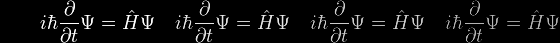# Eqn 11d Help

Suppose that p = exp (logeq) = e(raised to the power of logeq)

This would be a typographical nightmare … but there's a much simpler way of looking at it: the equation is telling us that the logarithm of p to the base e is logeq

 • logep = logeq

and in even simpler terms

 • p = q

So if we exponentiate both sides of x/a = loge {u + √(1+u2)}, we get

 • exp(x/a) = exp (loge {u + √(1+u2)}) which immediately simplifies to ex/a = u + √(1+u2)